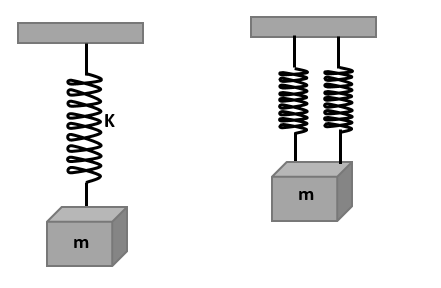Courses
Courses for Kids
Free study material
Free LIVE classes
More# A mass m performs oscillations of period T when hanged by spring of force constant K. If spring is cut in two parts and arranged in parallel and same mass is oscillated by them, then the new time period will beFigure: The spring-mass systemA. $2T$B. $T$C. $\dfrac{T}{{\sqrt 2 }}$D. $\dfrac{T}{2}$Verified
54.3k+ views
Hint: The spring constant of the spring is inversely proportional to the length of the spring. If the same spring is divided into smaller spring lengths then the resulting spring will have more stiffness than the original one. We find the equivalent stiffness of the combination then use it in the formula of the period of spring-mass system.

Formula used:
$T = 2\pi \sqrt {\dfrac{m}{k}}$
Where T is the period, k is the spring constant and m is the mass of the block.

Complete step by step solution:Figure: The spring-mass system
For the initial case, using period formula,
$T = 2\pi \sqrt {\dfrac{m}{k}}$

As we know that the spring constant of the spring is inversely proportional to the length of the spring, so when the spring is divided into two equal parts, then the spring constant of each equal parts will be twice of the original spring.

It is given that the spring constant of original spring is k, so the spring constant of each part will be 2k. Then, the equivalent spring constant in parallel is,
${K_{eq}} = 2k + 2k = 4k$

So, the final period of the system will be,
${T_2} = 2\pi \sqrt {\dfrac{m}{{4K}}}$
${T_2} = \dfrac{1}{2}\left( {2\pi \sqrt {\dfrac{m}{k}} } \right)$

On dividing the expression for the periods, we get
$\dfrac{T}{{{T_2}}} = \dfrac{{\left( {2\pi \sqrt {\dfrac{m}{k}} } \right)}}{{\dfrac{1}{2}\left( {2\pi \sqrt {\dfrac{m}{k}} } \right)}}$
$\dfrac{T}{{{T_2}}} = 2$
${T_2} = \dfrac{T}{2}$

So, the period of the new spring-mass system is half of the initial period.

Therefore, the correct option is (D).

Note: We should assume that the spring’s mass is negligible in relative to the mass suspended through the spring. Otherwise the motion of the block will not be perfect simple harmonic.
Last updated date: 23rd May 2023
Total views: 54.3k
Views today: 0.13k